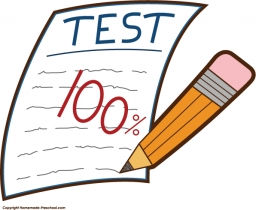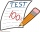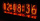# Quiz or test

I have a quiz with 20 questions. Each question has four multiple-choice answers, A, B, C, D. THERE IS NO WAY TO KNOW THE CORRECT ANSWER OF ANY GIVEN QUESTION, but the answers are static, in that if the "correct" answer to #1 = C, then it will always be equal to C. You can retake the quiz as many times as you want. You take the quiz the first time, making a log of all of your selections as you go (e.g., 1=C, 2=A, 3=D, 4=A, 5=A, 6=C, . . . . 19=A, 20=C). .. When complete, you learn you got 8 of 20 correct. So you take it again, and again log all of your answers, some different, some the same, and when done, you learn you got nine correct. You can retake it as often as you like. Using only guesses, what is the fastest way you could complete the test and get 100% correct, and what method would you use?

n =  4649045868

### Step-by-step explanation:Did you find an error or inaccuracy? Feel free to write us. Thank you!Tips to related online calculators
Do you want to convert time units like minutes to seconds?

## Related math problems and questions:

• Intelligence testPaľo, Jano, Karol, and Rišo were doing an intelligence test. Palo correctly answered half of the questions plus 7 questions, Jano to a third plus 18 questions, Karol to a quarter plus 21 questions and Risho to a fifth plus 25 questions. After the test, Ka
• The testThe test contains four questions, and there are five different answers to each of them, of which only one is correct, the others are incorrect. What is the probability that a student who does not know the answer to any question will guess the right answer
• Math testObelix filled a mathematical test in which he answered 25 questions. For every correct answer, he received 5 points, for each bad answer he had 3 points deducted. Obelix gained 36% of all points in the test. How many questions did he solve correctly?
• Six questions testThere are six questions in the test. There are 3 answers to each - only one is correct. In order for a student to take the exam, at least four questions must be answered correctly. Alan didn't learn at all, so he circled the answers only by guessing. What
• TestThe teacher prepared a test with ten questions. The student can choose one correct answer from the four (A, B, C, D). The student did not get a written exam at all. What is the probability that: a) He answers half correctly. b) He answers all correctly. c
• Petra's testThe answer will be given 8 marks, and the wrong answer will be deducted 3 marks. The total number of marks obtained by the school is 36. If Petra answered six questions correctly,   how many questions were answered incorrectly by the student from the scho
• Examination 2A teacher awards two marks for every correct answer and subtracts a mark for every wrong answer in an examination. If there are 20 questions and a child scored 25 marks, how many correct answers did the child get?
• Percentages above 100%What is 122% of 185? What is the meaning of percentages above 100%?
• ArelliArelli had 20 minutes to do a three-problem quiz. She spent 9 7/10 minutes on question A and 3 2/5 minutes on question B. How much time did she have left for question C? Solve on paper to find the answer as a fraction.After the history test, Michaella discovered that the ratio of her correct and incorrect answers is 5: 3. How many correct answers did Michaella have in the test, if she had 6 incorrect answers?
• GranddaughterIn 2014, the sum of the ages of Meghan's aunt, her daughter and her granddaughter was equal to 100 years. In what year was the granddaughter born, if we know that the age of each can be expressed as the power of two?
• TV competitionIn the competition, 10 contestants answer five questions, one question per round. Anyone who answers correctly will receive as many points as the number of competitors answered incorrectly in that round. After the contest, one of the contestants said: We
• EmilyEmily had 20 minutes to do a three-problem quiz. She spent 11 3/4 minutes on question A and 5 1/2 minutes on question B. How much time did she have left for question C?
• MegapizzaMegapizza will be divided among 100 people. First gets 1%, 2nd 2% of the remainder, 3rd 3% of the remainder, etc. Last 100th 100% of the remainder. Which person got the biggest portion?
• Percents from percentHow much is 13% of 20% of 500 greater than 8% of 14% of 200?
• Test scoresJo's test scores on the first four 100 point exams are as follows: 96,90,76, and 88. If all exams are worth the same percent, what is the minimum test score necessary on his last exam to earn an A grade in the class (90% or better)?
• Exchange € 100Find out how many ways you can exchange € 100 if you have an unlimited number of 50, 20, 10 and 5 euro banknotes. Use a method other than listing all options systematically.